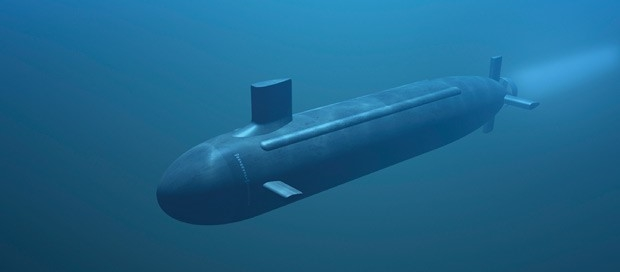# Learning Python with Advent of Code Walkthroughs

Dazbo's Advent of Code solutions, written in Python# Advent of Code 2021 - Day 2

## Problem Intro

We need to work out how to pilot the submarine! The input is a set of instructions that look like this:

``````forward 5
down 5
forward 8
up 3
``````

## Solution

### Setup

Similar to before:

``````import logging
import os
import time

SCRIPT_DIR = os.path.dirname(__file__)
INPUT_FILE = "input/input.txt"
# INPUT_FILE = "input/sample_input.txt"

logging.basicConfig(format="%(asctime)s.%(msecs)03d:%(levelname)s:%(name)s:\t%(message)s",
datefmt='%H:%M:%S')
logger = logging.getLogger(__name__)
logger.setLevel(level=logging.DEBUG)
``````

I’ve removed the date component from the logging.

### Part 1

We need to determine the horizontal position and depth after following the instructions, and then return the product of these two numbers. We start at horizontal position 0 and depth 0.

Again, pretty simple. We just need to track our horizontal position and depth with each instruction. So we need an x,y coordinate system. For this, tuples will do just fine. But I quite like using complex numbers, since it’s easy to add them, multiply them, whatever.

A complex number is made up of two parts: the `real` part and the `imaginary` part.Here, I’m using the real part to be the horizontal position, and the imaginary part to be the depth.

Let’s start by creating vectors that we can reuse:

``````VECTORS = {     # using complex numbers means we can track horizontal (real) and depth (imag) in one variable
'forward': 1+0j,    # increase horizontal
'down': 0+1j,       # increase depth (vertical)
'up': 0-1j,         # decrease depth
}
``````

Now read the instructions and process them:

``````    input_file = os.path.join(SCRIPT_DIR, INPUT_FILE)
with open(input_file, mode="rt", encoding="utf-8") as f:
data = [line.split() for line in f.read().splitlines()]

# Let's get tuples of instruction:str, instruction_magnitude:int
instructions = [(instr, int(instr)) for instr in data]

# Part 1
current_location = 0+0j
for instruction in instructions:
current_location += instruction*VECTORS[instruction]

logger.info("Part 1: Final location=%s", current_location)
logger.info("Location product = %d", current_location.real*current_location.imag)
``````

Here we’re using Python’s List Comprehension to read each line in the input data, and then split each line at the spaces. Thus, we end up with a list of pairs, i.e. instruction, magnitude. But both are type: `str`. We then perform another round of `list comprehension` to turn the magnitudes into type `int`.

We set our starting position `0+0j` and then iterate through the instructions. With each instruction, we obtain the relavent vector, and then we multiply that vector by the magnitude.

E.g. `down 3` would become:
`3 * 0+1j = 0 + 3j`

Add that to our current location.

Finally, return the product of the final location, as required. Easy!

### Part 2

Now we also need to track `aim` (i.e. inclination). We’re told that the `up` and `down` instructions now adjust the aim, rather than changing our depth.

So, we need a variable to store the aim, and we need to reset our starting position.

• For each up/down instruction, we now adjust the aim by the magnitude in the instruction.
• For each forward instruction, we increase the horizontal (real part) by the magnitude, and we increment the depth (imaginary part) by the aim multiplied by the magnitude.
``````   # Part 2
aim = 0 # track the inclination of the sub, in terms of depth change per unit horizontal
current_location = 0+0j
for instr, instr_mag in instructions:
if instr == 'down':
aim += instr_mag
elif instr == 'up':
aim -= instr_mag
else:
current_location += complex(instr_mag, instr_mag*aim)

logger.info("Part 2: Final location=%s", current_location)
logger.info("Location product = %d", current_location.real*current_location.imag)
``````

The result looks something like this:

``````17:16:33.494:INFO:__main__:     Part 1: Final location=(2034+702j)
17:16:33.495:INFO:__main__:     Location product = 1427868
17:16:33.495:INFO:__main__:     Part 2: Final location=(2034+770963j)
17:16:33.495:INFO:__main__:     Location product = 1568138742
17:16:33.495:INFO:__main__:     Execution time: 0.0014 seconds
``````

That’s it!